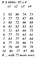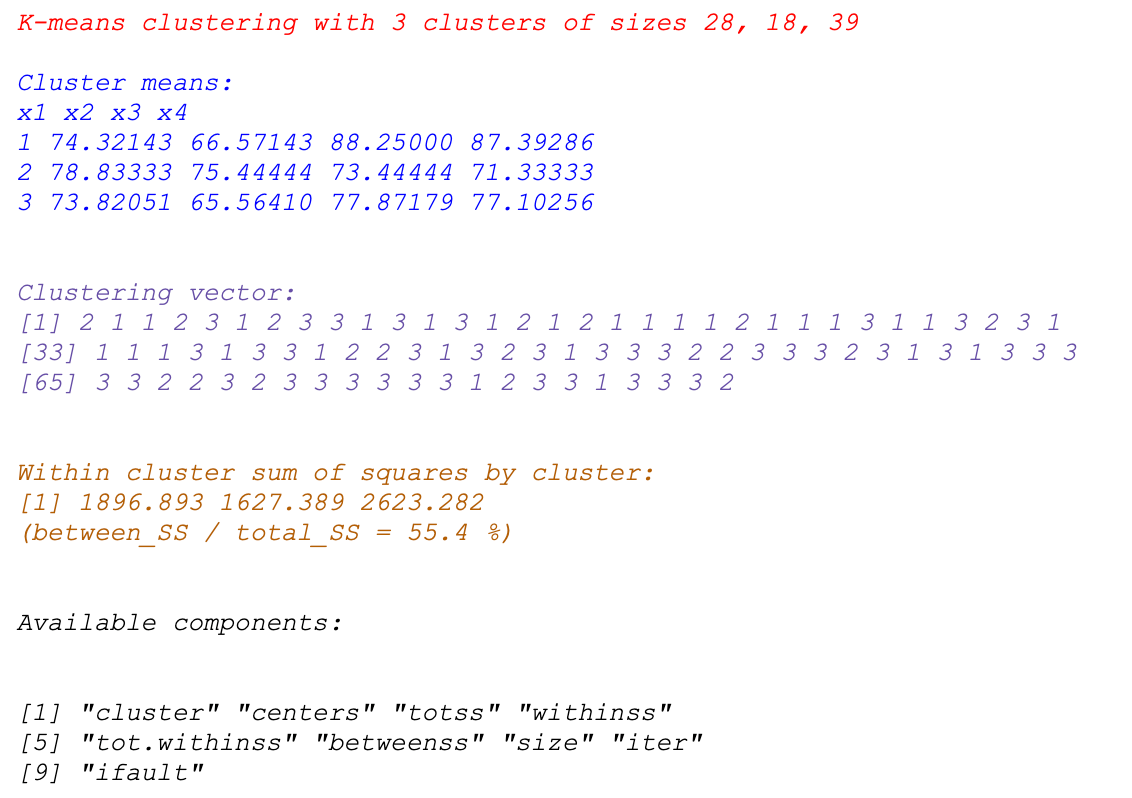K Means parameters and results (in R Studio) explained

Preliminary note: This post is the result of personal study on the subject. I studied computer science years ago at Politecnico of Milano university but data, especially business intelligence, became my profession. Data science has been the next step even if my background is more on programming and IT. I realise that there’s a lot to learn in this field so I tried to write with a beginner/student approach. Any suggestion for improvement is appreciated.

The objective of this article is explaining the results of the K Means algorithm that you get when you run it on your data using R Studio. I think that most of the comments I wrote can be applied to whatever tool you use for K Means:

• First of all you know that you use K Means algorithm to try to segment data in a number of clusters.
• To use any algorithm of this kind you have to provide that as input together with other parameters. To keep it simple I will use the algorithm with the minimum parameters to make it working because as said I want to explain the data in output.
• Let’s suppose you have 4 columns of a already prepared csv data set representing feature x1 to feature x4. Assume the values are separated by semicolons so you use the read_delim function instead of read_csv, and assign the results to variable ‘a’ in my case.A very easy csv file with random data (in range 0..99) organized in rows (observations) and columns (features). I have skipped the part on how you read it as I assume this is an easy task.
• Because you have to provide as input the number of clusters you want to find, you can try to visualise the data in advance using any plot function to check if the number of cluster (k) are someway visible so that you can do an educated gues. Alternatively you can use a number (like 3 for example) you think could be the outcome of the algorithm.

Using K Means is straightforward, you can check the RStudio help but basically the command is like the single line below with ‘a’ as my variable with the data set, 3 as number of clusters and the 2 params iter.max and nstart which I explain later.

• The results of the algorithm goes to variable ‘cl’ which I created with that name but could be what you prefer:
• cl<-kmeans(a,3, iter.max=50,nstart=10)

Let’s focus now on iter.max and nstart parameter which are apparently easy to understand but require attention:

• iter.max is the number of times the algorithm is run before results are returned. Do not forget that the algorithm works finding a minimum of a cost function through several iteration steps. If you say iter.max=1 it could work but it’s a nonsense as for the most times 1 iteration is not enough.
• On each step the algorithms compares the current value of the cost function (*) with the previous until the difference is considered “small”. If you specify for example 10 iterations it means that it gets the min after ten times the algorithm is run. it could be ok or it could not be ok depending on the data set. I found for example in simple data sets that it found the min after 1 iteration only even if I said iter.max=10, while in other cases even if you enter 100 iterations the algorithms oscillates around a local min and it never gets stable. A good number could be around 10–20 but you have to try and see the results.
• nstart represents the number of random data sets used to run the algorithm. It means that in order to get the algorithm initialized you should feed the algorithm with the initials coordinates of the cluster centers which you don’t want to do manually. So nstart extract for a number of times (example nstart=10) k random numbers between 1 and the number of “observations” in your csv file (the number of lines) and it takes that line as a starting point for cluster 1 up to cluster k candidates centroids. So if you want 3 clusters and you specify nstart=10 it extracts 3 sets of data, 10 times, and for each of these times, the algorithm is run (up to iter.max # of iterations) and the cost function is evaluated so that the lowest is then chosen as the result. In the above example is 10 times 50 = 500. As said this parameter does not guarantee that the minimum is always found but at least it should improve the results.
• (*) the cost function in kmeans is the total sum of the squares. In R Studio this is the variable \$totss which is accessible through the variable ‘cl’ in my example above: cl\$totss. The meaning of “total sum of squares” in kmeans is explained ahead.
• So you prepared the data in csv, loaded it and you run the algorithm… so what :-) ?

If you did things rights you should obtain something like this below out of RStudio:

• Let’s see the meaning of everything because we don’t want to use visualization but understanding the outputs :Sample of output of the Kmeans algorithm run in Rstudio. The objective here is not showing visually the results but explaining the terms the algorithm returns
• Title (in red): the output says we have our 3 clusters where the size of each cluster is made of 28,18,39 observations. In itself this is good to verify the homogeneous distribution of data for each cluster. So it’s a good start.
• Cluster means (in blue): these are the coordinates of the centroids. “Centroid” is the position of the cluster center which is obtained by minimising iteratively the sum of squares of the distances of the points with the “moving” point that, in the last step of the iteration, become the centroid of that cluster.
• Clustering Vector (in purple): this is the vector that tell us that each line of the csv file (observations) has been assigned to a cluster. so line 1 with observations x1..x4 to cluster 2, line 2 to cluster 1 and so on….
• Within clusters…(in orange): this part tell us the sum of squares within each cluster. we have 3 cluster so we have 3 numbers. it’s a vector and it can be accessed with the command cl\$withinss. This numbers mean that if you imagine a cluster like a cloud of points that have a distance from the centroid, this represents the sum of squares of the distances of the points assigned to a cluster from the centroid of that cluster.
• In this case the points in cluster 1 have a sum of square distances from the centroid equal to 1896.893, the points in cluster 2 have a sum of square distances from the centroid of 1627.389, the points in cluster 3 have a sum of square distances from the centroid of 2623.282.
• What does it mean ? It depends but lower these values are and more compact or dense is the cloud of points around its centroid. The ideal is when we have compact clusters well separated from each other that translates in the ratio (see ahead) of 100% in the ratio below.
• It’s important to understand the ratio betweenss / totss:
• betweenss (B) is the average of the square distances between the centroids. if you have k=3 clusters centroids, then you have to compute the average of (k*(k-1))/2 numbers representing the distances between the centroids. The divide by 2 is because the distances are all duplicated in the k x k matrix. So for example if k=3, you have to sum the square distances between c1 and c2, c1 and c3, c2 and c3 and divide the result by 3.
• tot.withinss (A) is the total sum of the squares distances in all the clusters of the points (observations) from the centroid to which that point is assigned. tot.withinss = sum(withinss). So if you have 3 clusters you will have 3 groups of “withinss” numbers that you have to sum together.
• totss (total_SS) is computed as the sum of \$tot.withinss (A)+ \$betweenss (B)
• So the ratio above (between_SS / total_SS = 55.4 %) is like (B / (A+B)) . you can see yourself that as much A (tot.withinss) tends to be small , the ratio tends to 1 (or 100%).
• It means that as much the points in each cluster are compact (low withinss for each cluster and therefore low tot.withinss which is the sum of all the withinss ) the better the clustering is because you have low variance in each cluster, in this case the separation of centroids (betweenss) explains the separation of the clusters which are compact and well separated.
• On the opposite if you have a high A (high withinss) then it could mean that one or more or all the clusters are sparse; so you have a high variance; above ratio is low. In a worst case scenario if your ratio is 0% it means that the clustering has not performed well at all.
• Having in my case 55% it’s not so good even if I have not seen any plot.
• It tells me that I could have points in clusters which are here and there and not well separated. This is also confirmed by the centroid coordinates above which you can see are not so different from each other. Of course here it comes the analysis part in which we have to evaluate the data and the relevance of the clusters numbers that we have found. So visualization could also help.
• part in black: these are simply variables names that bring us all the information resulted from running the algorithm. so for example cl\$clusters it give us back the part in red.

Well this completes the analysis of what RStudio outputs when we run K Means.

It is just the beginning of a journey but it’s a start. This is my first topic on this complex subject and I hope to have interested you.

IT Senior Manager and Consultant. Data Warehouse and Business Intelligence expertise in design and build. Freelance.

More from Andrea Grianti

IT Senior Manager and Consultant. Data Warehouse and Business Intelligence expertise in design and build. Freelance.